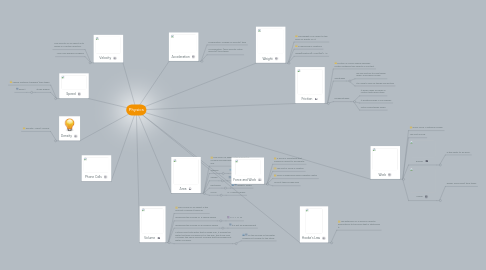# Physics

Get Started. It's FreePhysics## 1. Work

### 1.3. Energy

1.3.1. is the ability to do work.

### 1.4. Power

1.4.1. power: work done/ time taken

## 3. Friction

### 3.1. Friction is a force Which opposes motion between two objects in contact.

3.2.1. We use friction to slow things down: e.g brakes on bike

3.2.2. it is used to pick up things and writing

3.3.1. It wears away surfaces in contact with each other

3.3.2. It wastes energy in car engines

3.3.3. Often slows things down.

## 6. Area

### 6.2. Triangle

6.2.1. 1/29(base) × height

### 6.3. Square

6.3.1. length × length

### 6.4. Rectangle

6.4.1. lenght × width

## 7. Volume

### 7.2. measuring the volume of a regular shape

7.2.1. V= L × L× W

### 7.3. measuring the volume of an irregular shape

7.3.1. it is got by displacement

### 7.4. a stone is put into water that is inside a jar, it pushes the water that was occupied out of the way, the stone now occupies the same amount of space that the pushed out water occupied.

7.4.1. So the volume of the water pushed out is equal to the stone.

8.2.1. pg.261